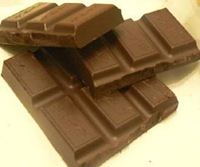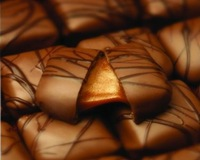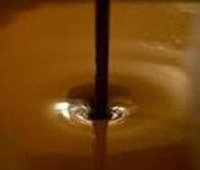Jul 09

## Guess Your Age by Chocolate

Here is one of those math tricks that has been floating around the Internet.  Don’t cheat by scrolling down!  It takes less than a minute.  At the end, I will provide an explanation of how this trick is done.Step 1:  Pick the number of times a week that you like to eat chocolate (more than once but less than 10).Step 2:  Multiply this number by 2 (just to be bold)!Step 3:  Add 5 to the total.Step 4:  Multiply your total by 50 — I’ll wait while you get a calculator.Step 5:  If you have already had your birthday this year, add 1759.  If you haven’t, then add 1758.Step 6:  Now subtract the four digit year that you were born.Your result should be a three digit number. The first digit is your original number (i.e., how many times you want to have chocolate each week).  The next two numbers are your age!

This is the only year (2009) that this trick will work, so spread it around!

### How to Solve this Trick

This is a simple algebra math trick.  For test purposes, let’s assume you were born on January 1, 1970.  That would make your age 39 (as of today, July 9, 2009).  So, following the steps above:

1. Pick the number of times a week that you like to eat chocolate.  So let’s assign the variable “C” for chocolate:
= C

2. Multiply this number by 2.  So that’s:
= C x 2

3. Add 5 to the total.
= (C x 2) + 5

4. Multiply your total by 50.
= ((C x 2) + 5) x 50
= (C x 2 x 50) + (5 x 50)
= (C x 100) + 250

= ((C x 100) + 250) + 1759
= (C x 100) + 2009 (which is the current year!)

6. Subtract the four year digit you were born.
= (C x 100) + 2009 – 1970
= (C x 100) + 39 (which is our test age!)
= C39

The final result is a three digit number.  The first digit is your original number “C” (C x 100).  The next two numbers are your age (39).

So the trick is to take your original number and multiply it by 100 so that it’s the first digit in the result.  Next, it multiplies and adds some numbers that equal the current year.  So then if you subtract the year you were born, the result is the number of years you have been alive, i.e., your age.

SOLVED!

If you enjoyed this puzzle, be sure to check out the Regifting Robin Mind Trick.

Article published on July 9, 2009

### 11 Responses to “Guess Your Age by Chocolate”

1. Ian Says:

Tried 3 times and didn’t work for me – number was 5 digits long.

2. KIMBERLEY Says:

ACE I DID IT IN MY MATHS LESSON 2DAY A TRIED IT ON MY MUM AND DAD IT IS THE GREASTING THING I HAVE EVER SEEN!!!!!!! <3

3. freya Says:

this is so cool how do u do it

4. Shelley Says:

That is so cool. It worked everytime. And, Freya, it says how to do it!

5. cerysnapril Says:

hahahahahahahahahahaha it doesn’t work me and April tried it it said we were 11 in fact people we are 12 hahahahahahahahahaha RUBBISH !!!!!!!!

6. IZZY! Says:

It does not work it said i was 11 when i am twelve!

7. 555 Says:

I am 12 not 11!

8. Guess Your Age | More More Pics Says:

[…] Guess Your Age by Chocolate devtopics.com […]

9. Jen Says:

it said i was 12, im 14 :L

10. Fanny Says:

my number was 16….I’m 18… and i dont eat chockolate

11. Jack Says:

Why do I always see these “It didn’t work I’m x+1 or x+2 years old” .. read where it says it ONLY WORKS for 2009!! (or add 2 for 2011 to the equation and it will work)